## Example Questions

← Previous 1 3 4 5 6

### Example Question #1 : How To Simplify Square Roots

Simplify

÷ √3

3√3

not possible

2

3

none of these

3√3

Explanation:

in order to simplify a square root on the bottom, multiply top and bottom by the root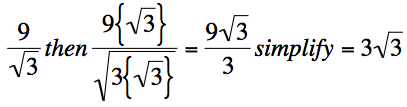### Example Question #2 : How To Simplify Square Roots

Simplify:

√112

12

4√7

20

10√12

4√10

4√7

Explanation:

√112 = {√2 * √56} = {√2 * √2 * √28} = {2√28} = {2√4 * √7} = 4√7

### Example Question #3 : How To Simplify Square Roots

Simplify:

√192

None of these
8√2
4√3
4√2
8√3
Explanation:

√192 = √2 X √96

√96 = √2 X √48

√48 = √4 X√12

√12 = √4 X √3

√192 = √(2X2X4X4) X √3

= √4X√4X√4  X √3

= 8√3

### Example Question #4 : How To Simplify Square Roots

What is the simplest way to express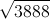?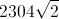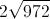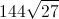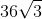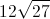Explanation:

First we will list the factors of 3888: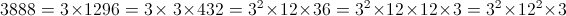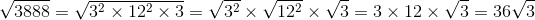### Example Question #5 : How To Simplify Square Roots

Simplify: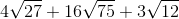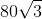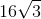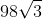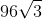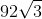Explanation:

4√27 + 16√75 +3√12 =

4*(√3)*(√9)  + 16*(√3)*(√25)  +3*(√3)*(√4) =

4*(√3)*(3) + 16*(√3)*(5) + 3*(√3)*(2) =

12√3  + 80√3 +6√3= 98√3

### Example Question #1 : How To Simplify Square Roots

Simplify the following: (√(6) + √(3)) / √(3)

3√(2)

1

√(3)

√(2) + 1

√(2) + 1

Explanation:

Begin by multiplying top and bottom by √(3):

(√(18) + √(9)) / 3

Note the following:

√(9) = 3

√(18) = √(9 * 2) = √(9) * √(2) = 3 * √(2)

Therefore, the numerator is: 3 * √(2) + 3.  Factor out the common 3: 3 * (√(2) + 1)

Rewrite the whole fraction:

(3 * (√(2) + 1)) / 3

Simplfy by dividing cancelling the 3 common to numerator and denominator: √(2) + 1

### Example Question #1 : How To Simplify Square Roots

what is

√0.0000490

0.007

7

0.00007

49

0.07

0.007

Explanation:

easiest way to simplify: turn into scientific notation

√0.0000490= √4.9 X 10-5

finding the square root of an even exponent is easy, and 49 is  a perfect square, so we can write out an improper scientific notation:

√4.9 X 10-5√49 X 10-6

√49 = 7; √10-6 = 10-3 this is equivalent to raising 10-6 to the 1/2 power, in which case all that needs to be done is multiply the two exponents: 7 X 10-3= 0.007

### Example Question #8 : How To Simplify Square Roots

Simplify: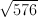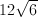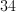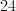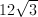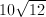Explanation:

In order to take the square root, divide 576 by 2.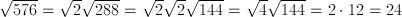### Example Question #1 : How To Simplify Square Roots

Simplify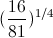.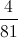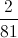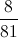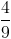Explanation: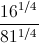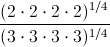### Example Question #21 : Arithmetic

Simplfy the following radical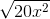.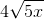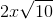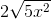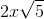Explanation:

You can rewrite the equation as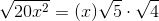.

This simplifies to.

← Previous 1 3 4 5 6

### All SAT Math Resources